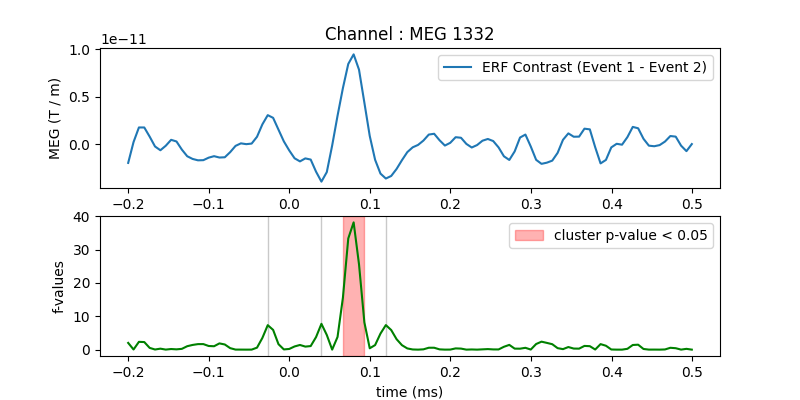# Permutation F-test on sensor data with 1D cluster level#

One tests if the evoked response is significantly different between conditions. Multiple comparison problem is addressed with cluster level permutation test.

```# Authors: Alexandre Gramfort <alexandre.gramfort@inria.fr>
#
```
```import matplotlib.pyplot as plt

import mne
from mne import io
from mne.stats import permutation_cluster_test
from mne.datasets import sample

print(__doc__)
```

Set parameters

```data_path = sample.data_path()
meg_path = data_path / 'MEG' / 'sample'
raw_fname = meg_path / 'sample_audvis_filt-0-40_raw.fif'
event_fname = meg_path / 'sample_audvis_filt-0-40_raw-eve.fif'
tmin = -0.2
tmax = 0.5

#   Setup for reading the raw data

channel = 'MEG 1332'  # include only this channel in analysis
include = [channel]
```
```Opening raw data file /home/circleci/mne_data/MNE-sample-data/MEG/sample/sample_audvis_filt-0-40_raw.fif...
Read a total of 4 projection items:
PCA-v1 (1 x 102)  idle
PCA-v2 (1 x 102)  idle
PCA-v3 (1 x 102)  idle
Average EEG reference (1 x 60)  idle
Range : 6450 ... 48149 =     42.956 ...   320.665 secs
```

Read epochs for the channel of interest

```picks = mne.pick_types(raw.info, meg=False, eog=True, include=include,
event_id = 1
epochs1 = mne.Epochs(raw, events, event_id, tmin, tmax, picks=picks,
baseline=(None, 0), reject=reject)
condition1 = epochs1.get_data()  # as 3D matrix

event_id = 2
epochs2 = mne.Epochs(raw, events, event_id, tmin, tmax, picks=picks,
baseline=(None, 0), reject=reject)
condition2 = epochs2.get_data()  # as 3D matrix

condition1 = condition1[:, 0, :]  # take only one channel to get a 2D array
condition2 = condition2[:, 0, :]  # take only one channel to get a 2D array
```
```Not setting metadata
72 matching events found
Setting baseline interval to [-0.19979521315838786, 0.0] sec
Applying baseline correction (mode: mean)
4 projection items activated
Rejecting  epoch based on EOG : ['EOG 061']
Rejecting  epoch based on EOG : ['EOG 061']
Rejecting  epoch based on EOG : ['EOG 061']
Rejecting  epoch based on EOG : ['EOG 061']
Rejecting  epoch based on EOG : ['EOG 061']
Rejecting  epoch based on EOG : ['EOG 061']
Rejecting  epoch based on EOG : ['EOG 061']
Rejecting  epoch based on EOG : ['EOG 061']
Rejecting  epoch based on EOG : ['EOG 061']
Rejecting  epoch based on EOG : ['EOG 061']
Rejecting  epoch based on EOG : ['EOG 061']
Rejecting  epoch based on EOG : ['EOG 061']
Rejecting  epoch based on EOG : ['EOG 061']
Rejecting  epoch based on EOG : ['EOG 061']
Rejecting  epoch based on EOG : ['EOG 061']
Rejecting  epoch based on EOG : ['EOG 061']
73 matching events found
Setting baseline interval to [-0.19979521315838786, 0.0] sec
Applying baseline correction (mode: mean)
4 projection items activated
Rejecting  epoch based on EOG : ['EOG 061']
Rejecting  epoch based on EOG : ['EOG 061']
Rejecting  epoch based on EOG : ['EOG 061']
Rejecting  epoch based on EOG : ['EOG 061']
Rejecting  epoch based on EOG : ['EOG 061']
Rejecting  epoch based on EOG : ['EOG 061']
Rejecting  epoch based on EOG : ['EOG 061']
Rejecting  epoch based on EOG : ['EOG 061']
Rejecting  epoch based on EOG : ['EOG 061']
Rejecting  epoch based on EOG : ['EOG 061']
Rejecting  epoch based on EOG : ['EOG 061']
```

Compute statistic

```threshold = 6.0
T_obs, clusters, cluster_p_values, H0 = \
permutation_cluster_test([condition1, condition2], n_permutations=1000,
threshold=threshold, tail=1, n_jobs=None,
```
```stat_fun(H1): min=0.000227 max=38.167093
Running initial clustering …
Found 4 clusters

0%|          | Permuting : 0/999 [00:00<?,       ?it/s]
9%|8         | Permuting : 86/999 [00:00<00:00, 2500.43it/s]
17%|#7        | Permuting : 173/999 [00:00<00:00, 2530.84it/s]
28%|##7       | Permuting : 276/999 [00:00<00:00, 2707.87it/s]
41%|####      | Permuting : 407/999 [00:00<00:00, 2722.21it/s]
50%|####9     | Permuting : 497/999 [00:00<00:00, 2708.20it/s]
59%|#####9    | Permuting : 593/999 [00:00<00:00, 2730.97it/s]
68%|######8   | Permuting : 681/999 [00:00<00:00, 2709.32it/s]
77%|#######7  | Permuting : 773/999 [00:00<00:00, 2710.24it/s]
86%|########6 | Permuting : 860/999 [00:00<00:00, 2690.04it/s]
95%|#########5| Permuting : 954/999 [00:00<00:00, 2695.28it/s]
100%|##########| Permuting : 999/999 [00:00<00:00, 2695.54it/s]
100%|##########| Permuting : 999/999 [00:00<00:00, 2692.96it/s]
```

Plot

```times = epochs1.times
fig, (ax, ax2) = plt.subplots(2, 1, figsize=(8, 4))
ax.set_title('Channel : ' + channel)
ax.plot(times, condition1.mean(axis=0) - condition2.mean(axis=0),
label="ERF Contrast (Event 1 - Event 2)")
ax.set_ylabel("MEG (T / m)")
ax.legend()

for i_c, c in enumerate(clusters):
c = c
if cluster_p_values[i_c] <= 0.05:
h = ax2.axvspan(times[c.start], times[c.stop - 1],
color='r', alpha=0.3)
else:
ax2.axvspan(times[c.start], times[c.stop - 1], color=(0.3, 0.3, 0.3),
alpha=0.3)

hf = plt.plot(times, T_obs, 'g')
ax2.legend((h, ), ('cluster p-value < 0.05', ))
ax2.set_xlabel("time (ms)")
ax2.set_ylabel("f-values")
```Total running time of the script: ( 0 minutes 6.558 seconds)

Estimated memory usage: 9 MB

Gallery generated by Sphinx-Gallery